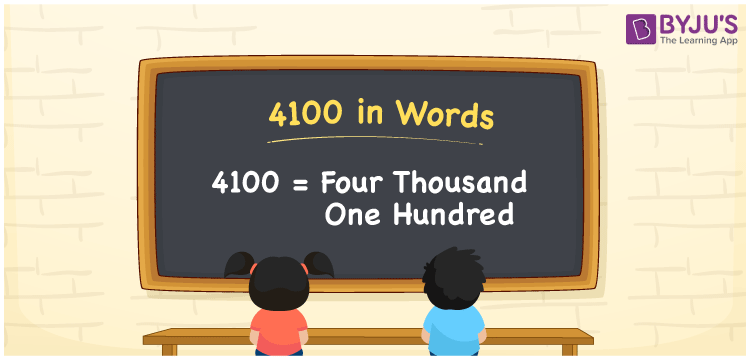# 4100 in Words

4100 in words can be written as Four Thousand One Hundred. Students will be able to learn the conversion of 4100 in words which will help them understand the applications of numbers in our daily lives. If you buy a grinder for Rs. 4100, then you can say that “I have bought a grinder for Four Thousand One Hundred Rupees”. The  number 4100 can be written in words using the English alphabet. The numbers in words can be grasped easily by the students using the resources given at BYJU’S. 4100 in English can be read as “Four Thousand One Hundred”.

 4100 in words Four Thousand One Hundred Four Thousand One Hundred in Numbers 4100

## 4100 in English Words## How to Write 4100 in Words?

Students will learn about the conversion of 4100 into words from place value charts. The number 4100 has four digits. For 4100, the place value chart is prepared in a table form to help students understand it effectively.

 Thousands Hundreds Tens Ones 4 1 0 0

4100 in expanded form is explained in brief here:

4 × Thousand + 1 × Hundred + 0 × Ten + 0 × One

= 4 × 1000  + 1 × 100 + 0 × 10 + 0 × 1

= 4000 + 100

= 4100

= Four Thousand One Hundred

Therefore, 4100 in words is written as Four Thousand One Hundred.

4100 is a natural number that precedes 4101 and succeeds 4099.

4100 in words – Four Thousand One Hundred

Is 4100 an odd number? – No

Is 4100 an even number? – Yes

Is 4100 a perfect square number? – No

Is 4100 a perfect cube number? – No

Is 4100 a prime number? – No

Is 4100 a composite number? – Yes

## Frequently Asked Questions on 4100 in Words

Q1

### How do you write 4100 in words?

4100 can be written as “Four Thousand One Hundred” in words.
Q2

### Is 4100 an even number?

4100 is an even number because it is divisible by 2.
4100/2 = 2050
Q3

### How can Four Thousand One Hundred be written in numbers?

Four Thousand One Hundred can be written in numbers as 4100.